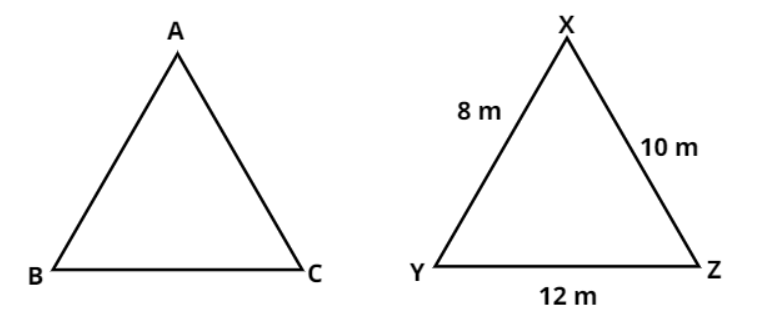Courses
Courses for Kids
Free study material
Free LIVE classes
MoreLIVE
Join Vedantu’s FREE Mastercalss

# For a $\vartriangle {\text{XYZ}}$, ${\text{ZY}} = 12{\text{ m}}$, ${\text{YX}} = 8{\text{ m}}$ and ${\text{XZ}} = 10{\text{ m}}$. If $\vartriangle {\text{ZYX}} \cong \vartriangle {\text{ABC}}$, then the length of side AC (in meters) is${\text{A}}{\text{. 10}} \\ {\text{B}}{\text{. 12}} \\ {\text{C}}{\text{. 8}} \\ {\text{D}}{\text{. 1}} \\$Verified
363k+ views
Hint- Here, we will be using the property that the length of the corresponding sides will be equal in any two congruent triangles.

Given, in a $\vartriangle {\text{XYZ}}$, ${\text{ZY}} = 12{\text{ m}}$, ${\text{YX}} = 8{\text{ m}}$ and ${\text{XZ}} = 10{\text{ m}}$
Since we know that for any two congruent triangles, the corresponding sides are always equal.
Also given that $\vartriangle {\text{ZYX}} \cong \vartriangle {\text{ABC}}$
Clearly from the figure, we can see that the side AB in $\vartriangle {\text{ABC}}$ is corresponding to side XY in $\vartriangle {\text{XYZ}}$, side BC in $\vartriangle {\text{ABC}}$ is corresponding to side YZ in $\vartriangle {\text{XYZ}}$ and side AC in $\vartriangle {\text{ABC}}$ is corresponding to side XZ in $\vartriangle {\text{XYZ}}$.
i.e., we can say ${\text{AB}} = {\text{XY}} = {\text{YX}} = 8{\text{ m}}$, ${\text{BC}} = {\text{YZ}} = {\text{ZY}} = 12{\text{ m}}$ and ${\text{AC}} = {\text{XZ}} = 10{\text{ m}}$.
Hence, the length of side AC is 10 meters.
Therefore, option A is correct.

Note- In these type of problems, the corresponding sides of the given congruent triangles are needed to be identified carefully with the help of their diagrams.

Last updated date: 16th Sep 2023
Total views: 363k
Views today: 5.63k